Request a number, for example: between 1 and 15, a time in the last 24 hours, or a day in the next month, using the different types of range questions.

We explain each option and how it looks in the mEMA App in this article.

1 Set Up

Each style of range question requires a minimum and maximum value.

Minimum Value: Here enter the lowest value that is allowable as an answer. In the example below the question asks for a number between 1 and 100 so in the minimum value field you would enter "1".

Maximum Value: Here enter the highest value that is allowable as an answer. In the example below the question asks for a number between 1 and 100 so in the maximum value field you would enter "100".

Question Editing WizardTypes of Range Questions

Details on each style of range question and how to use it.

Integer
Basic, whole number range question.
Disregard the "precision" value in this type.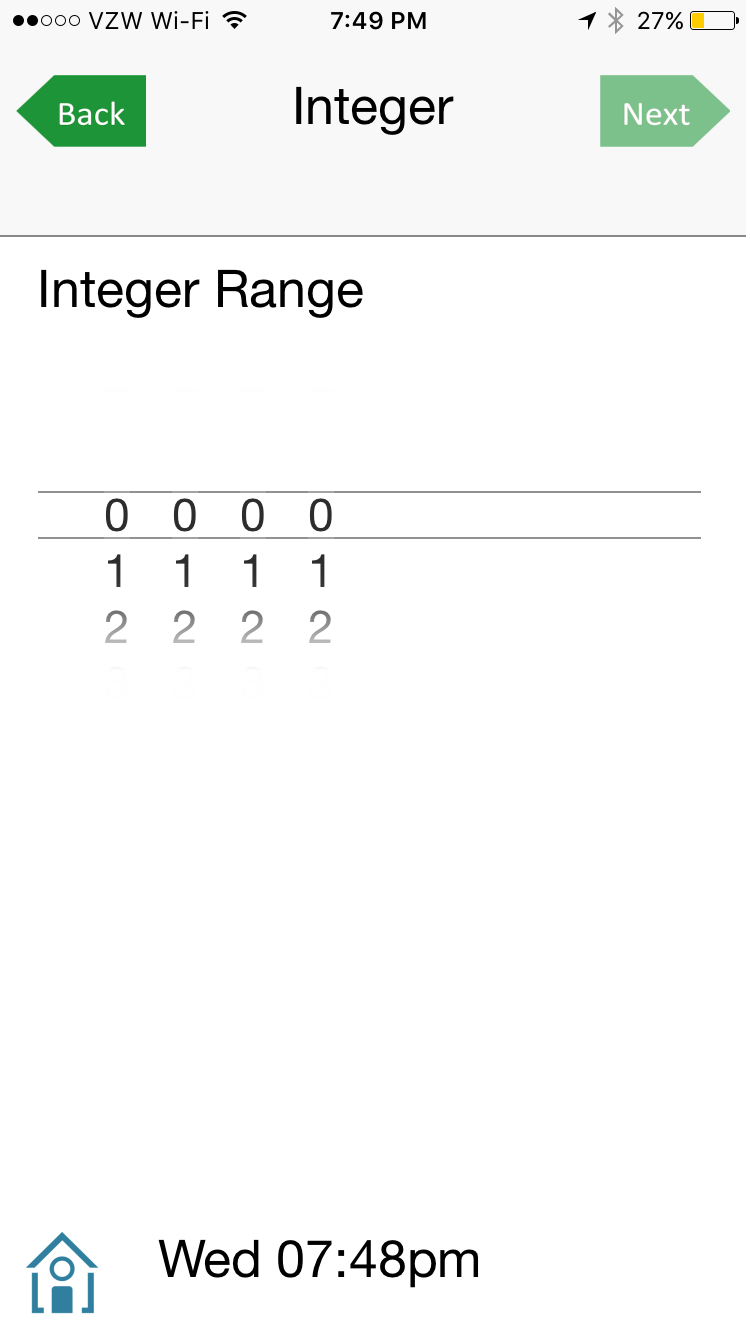Real Number
Allows for real numbers with decimals. Enter as many zeros as decimal places desired.
The "precision" box does not allow input, but will automatically populate.

In Survey EditorIn mEMA App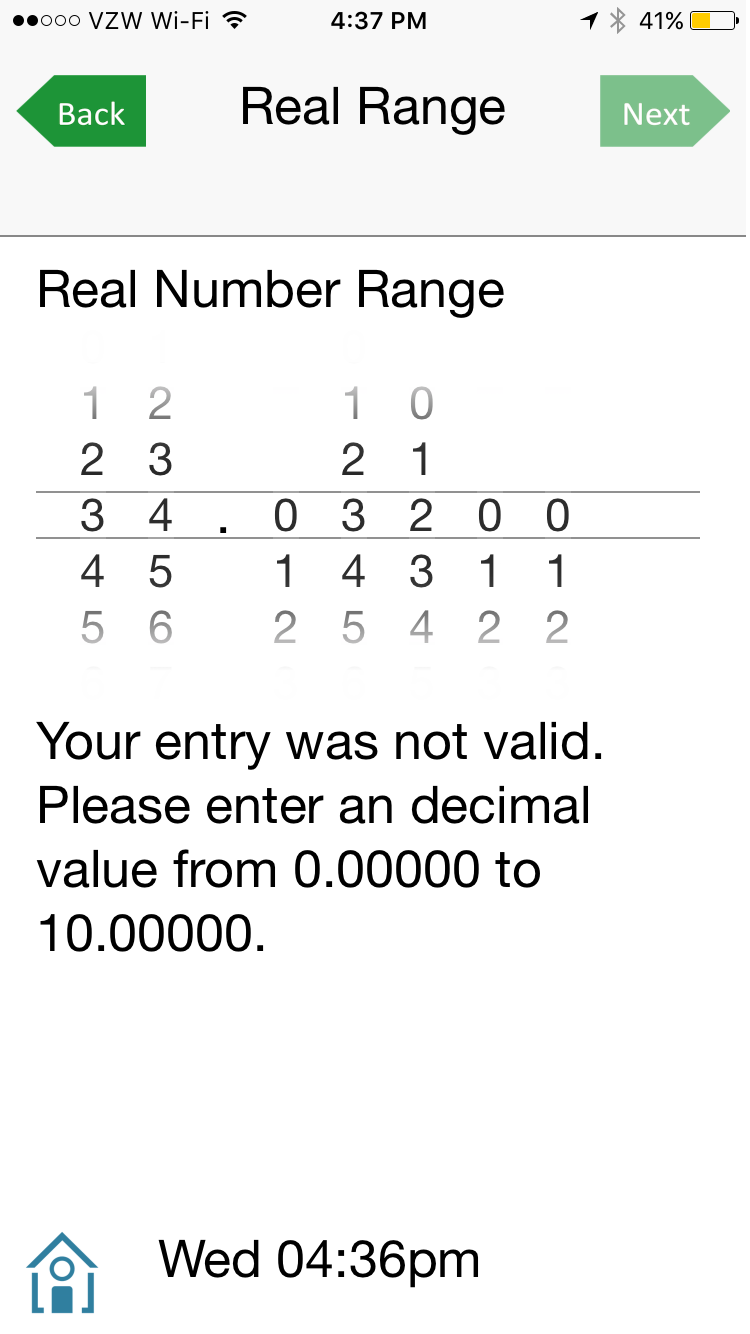Date

Asks for a date between two set dates. The box will automatically format the date correctly.

Disregard the "precision" value in this type.

In Survey Editor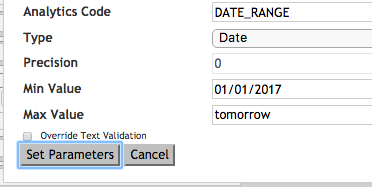In mEMA AppDate/Time

Asks for both a date and a time.

This option can be set to reflect the current date and time by entering "now" "yesterday" or "tomorrow".

Disregard the "precision" value in this type.

In Survey EditorIn mEMA AppDuration Range

Allow the respondent to enter an amount of time. Specify the units of measurement and potential range.

We recommend selecting up to three units of time measurement, more will not fit on most phone screens.

In Survey Editor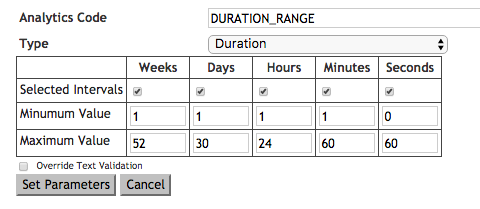In mEMA AppUse answers to previous questions as the parameters for the next question.

Set this question up in the same way one would a dependency. Enter, within brackets, the question numbers whose answers you want use as the range parameters. It is necessary to use the same unit (integer for example) for all the questions involved in this type of range question.

Disregard the "precision" value in this type.

In Survey Editor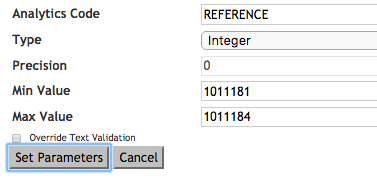In mEMA App